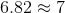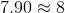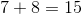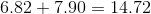# ISEE Lower Level Math : How to add

## Example Questions

← Previous 1 3 4 5 6

### Example Question #1 : How To Add

Thomas rides the bus to school each morning. If the bus picks Thomas up at a quarter before eight, and it takes half an hour to get to school, what time will Thomas arrive at school?

a quarter before 9

a quarter after 8

8 o'clock

half past 8

half past 9

a quarter after 8

Explanation:

The phrase 'a quarter before' indicates that the time is 15 minutes before the hour.

The bus picks Thomas up at

"a quarter before 8"

which translates to 15 minutes before 8 o'clock, or

7:45 AM.

Because it takes half an hour, or

30 minutes

to get to school, Thomas will arrive at school 30 minutes after 7:45 AM.

7:45 AM + 30 minutes =

8:15 AM

'a quarter after' indicates that the time is 15 minutes after the hour, so 8:15 can be read as

a quarter after 8.

Note that there are 60 minutes in one hour.

### Example Question #1 : Addition And Subtraction With Money

Approximately how much will John need to pay if a pair of shoes cost $42.64, a pair of pants costs$37.99, and a shirt costs $21.34. Possible Answers:$120

$110$80

$100$90

$100 Explanation: The key word, "approximately," indicates that we must round each of these values. Let's round to the nearest tens place because each of our answer choices is rounded to the tens place. Shoes cost$42.64.

$42.64 rounded (down) to the nearest tens place is$40.

Pants cost $37.99.$37.99 rounded (up) to the nearest tens place is $40. A shirt costs$21.34.

$21.34 rounded (down) to the nearest tens place is$20.

Let's add up our estimated prices.

$40 +$40 + $20 =$100.

### Example Question #181 : Operations

Evaluate: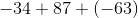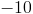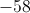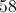Explanation:

Add the first two numbers as follows: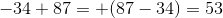Now add this sum to the third number as follows: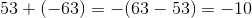### Example Question #1 : How To Add

Evaluate: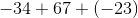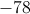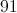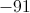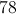Explanation:

Add the first two numbers as follows: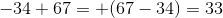Now add this sum to the third number as follows: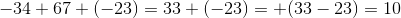### Example Question #1 : How To Add

Add. Show as a mixed fraction.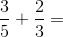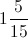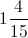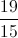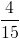Explanation:

When adding fractions, you must first make sure that the denominators are equal. If they are not equal, then you must make them equal by finding the LCD (lowest common denominator). In this case the LCD is 15. Now set up the new equation: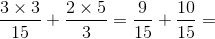Then add the numerators to get: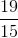Show as a mixed fraction: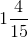### Example Question #1 : Addition And Subtraction With Money

Approximately how much will Sophie need to buy a shirt at $12.99, a loaf of bread at$3.99, and a television at $43.76? Possible Answers:$100

$80$61

$60 Correct answer:$61

Explanation:

Since we're looking for an approximate value, we need to round our numbers up. Adding the three items gives a total of $60.74, so at least$61 is needed - thus $61 is the correct answer. ### Example Question #13 : Money And Time Laura is going bowling with her friends this weekend and she is using some of the money that she has saved up from allowance and her birthday. She will have to pay a flat fee of$8 to rent her shoes and $10.50 per game she bowls. If she has planned to bowl 4 games and she is only taking$50 with her to the bowling alley will she have enough money?  If yes, how much money will she have when she leaves the bowling alley?

No

Yes,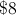Yes,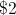Yes, None.

Yes, None.

Explanation:

The flat fee for the shoes will only be paid once since the shoes will be used for all four games.  The cost per game will be paid each time one game finishes and a new one is started.

To calculate her cost add the fee for the shoes and the fee for each game played.  That would be $8 (shoes) +$10.50 (game 1) + $10.50 (game 2) +$10.50 (game 3) + $10.50 (game 4) =$50 (total cost).

Laura had \$50 with her so there was enough money and nothing left over when she left the bowling alley.

### Example Question #1 : Addition And Subtraction With Money

Danielle buys four items costing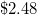,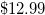,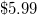, and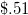. What is the estimated total of Danielle's items?

Between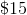and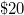Betweenand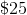Betweenand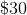Betweenand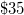BetweenandExplanation:

To solve this problem, one should round either to the tenths place, or to the nearest dollar.can be rounded to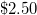,to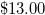,to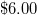, andto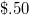. When one adds up the estimated costs, it yields about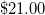.

### Example Question #1 : Addition And Subtraction With Money

Sam buys four items, costing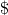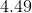,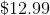,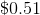and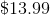. What is the estimated cost of Sam's items?

Betweenanddollars

Betweenanddollars

Betweenanddollars

Betweenanddollars

Betweenanddollars

Explanation:

In this problem, the costs of the items can be rounded to either the nearest dollar, or the nearest ten cents.andcan be rounded toand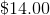, respectively.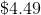can be rounded to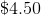, andcan be rounded to.

When the new, estimated costs of the items are added, the sum is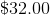, which is betweenanddollars.

### Example Question #2 : How To Add

Which of the following is a valid approximation of the problem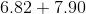?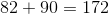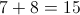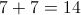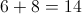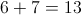Explanation:

Round each number to the nearest whole number: